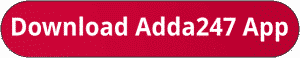Latest Banking jobs   »   Bank Foundation quant   »   Bank Foundation quant

# Quantitative Aptitude Quiz For Bank Mains Exam 2023-21th November

Q1. Quantity I: Height of the tank if the volume of cylindrical tank is 12320 cubic cm. Its radius and height are in the ratio of 7 : 10 respectively.
Quantity II: Level kerosene in the jar. A conical vessel of base radius 2 cm and height 3 cm is filled with kerosene. This liquid leaks through a hole in the bottom and collects in a cylindrical jar of radius 2 cm.

(a) Quantity I > Quantity II
(b) Quantity I < Quantity II
(c) Quantity I ≥ Quantity II
(d) Quantity I ≤ Quantity II
(e) Quantity I = Quantity II or No relation

Q2. A man who swim 48m/minute in still water, swims 200m against the current and 200m with the current. The difference between the time taken by him against the stream and with the stream is 10 minutes.
Quantity I: speed of current.
Quantity II: Speed of a man who completes 3 rounds of a circular path of radius 49 m in 14 minutes.

(a) Quantity I > Quantity II
(b) Quantity I < Quantity II
(c) Quantity I ≥ Quantity II
(d) Quantity I ≤ Quantity II
(e) Quantity I = Quantity II or No relation

Q3. Quantity I: Marked price (in rs.) of an article if a tradesman gives 4% discount on the marked price and 1 article free with every 15 articles bought and still gains 35%. C.P. of the article is Rs. 100.
Quantity II: Profit (in rs.) made on selling the pen at marked price. Even after reducing the marked price of a pen by Rs. 32, a shopkeeper makes a profit of 15%. The cost price of a pen is Rs. 320.
(a) Quantity I > Quantity II
(b) Quantity I < Quantity II
(c) Quantity I ≥ Quantity II
(d) Quantity I ≤ Quantity II
(e) Quantity I = Quantity II or No relation

Q4. Quantity I – How many three digits positive integers, with digits x, y and z exist such that x < y and z < y (Assume that ‘x’ is in hundred’s place, ‘y’ is in ten’s place and ‘z’ is in unit’s place).
Quantity II – Set A comprises all multiples of four less than 500, set B comprises all odd multiples of seven less than 500 and Set C comprises all multiples of six less than 500. How many elements are present in (A ∪ B ∪ C)
(a) Quantity I ≥ Quantity II
(b) Quantity I < Quantity II
(c) Quantity I > Quantity II
(d) Quantity I ≤ Quantity II
(e) Quantity I = Quantity II or No relation

Directions (5-5): The following questions are accompanied by two statements I and II. You have to determine which statements(s) is/are sufficient/necessary to answer the questions.

Q5. Find the sum of present age of X & Z?
I. Four years ago, average age of X, Y and Z was 16 years while age of X, Y and Z is in arithmetic progression.
II. Average of present age of X and Z is same as present age of Y while Y is four years younger than Z.
(a) Statement I alone is sufficient to answer the question but statement II alone is not sufficient to answer the question.
(b) Statement II alone is sufficient to answer the question but statement I alone is not sufficient to answer the question.
(c) Both the statements taken together are necessary to answer the questions, but neither of the statements alone is sufficient to answer the question.
(d) Either statement I or statement II by itself is sufficient to answer the question.
(e) Statements I and II taken together are not sufficient to answer the question.

Solutions.                                                           .                                           .      .             .

## FAQs

### When is the IBPS Clerk Mains exam scheduled for?

The IBPS Clerk Mains exam is scheduled for 7 October 2023.

#### Congratulations!Union Budget 2023-24: Free PDF